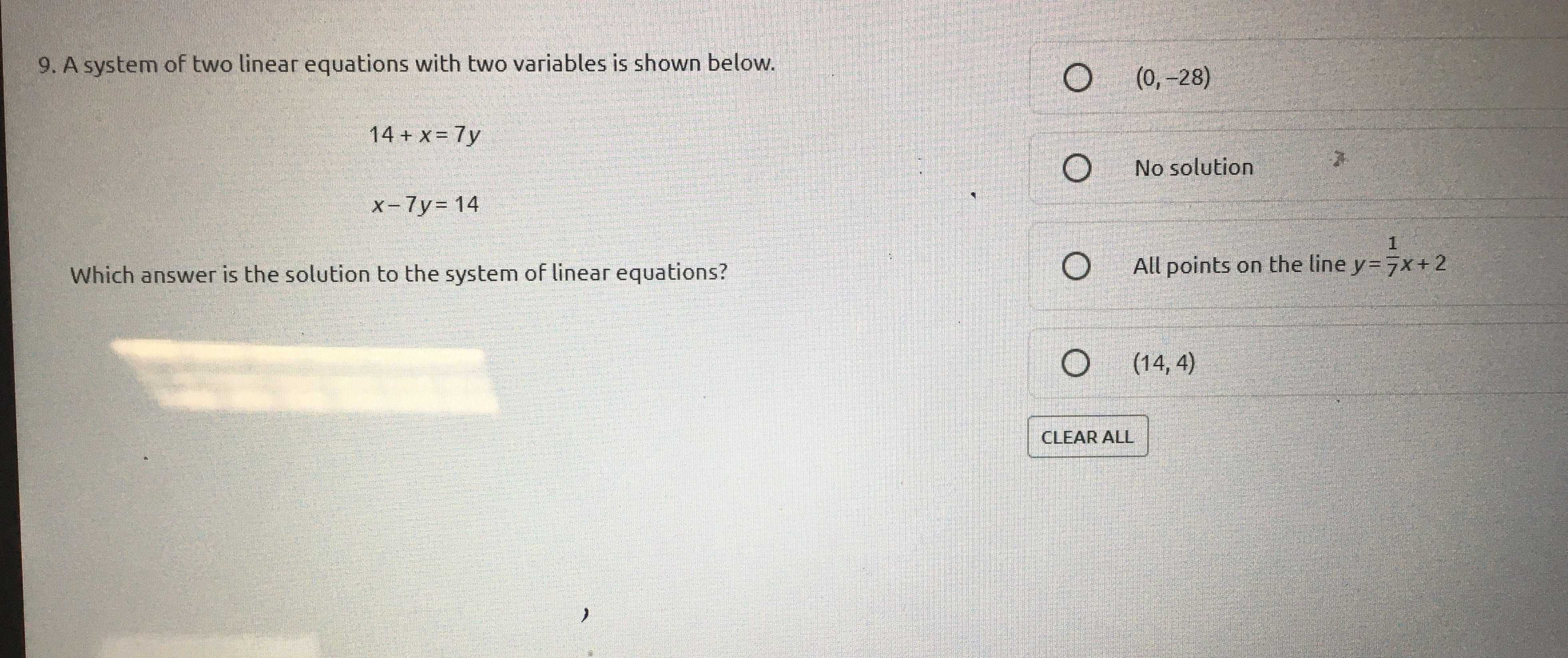### Still have math questions?

Algebra
QuestionA system of two linear equations with two variables is shown below.

$$14 + x = 7 y$$ $$x - 7 y = 14$$

Which answer is the solution to the system of linear equations?

$$\square$$$$( 0 , - 28 )$$

$$\square$$No solution

$$\square$$All points on the line $$y = \frac { 1 } { 7 } x + 2$$

$$\square$$$$( 14,4 )$$